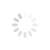## ABCD patternOne of the most basic harmonic patterns – ABCD pattern. Here is how it works.

You have AB and CD in the picture. These are called legs of the pattern. BC movement is a retraction. In this case AB and CD are always going to be of equal length as well as the amount is time it takes to go from A to B is going to be the same as from C to D.

This pattern can be used to trace trend overturn using Fibonacci retracement. Here Fibonacci retracement on AB, the retracement BC should reach 0.618 level. Next, the line CD should be the 1.272 Fibonacci extension of BC. You can also use the percentage stated in the picture for calculations although it is better to simply use the rules marked here with RED.

## 222 pattern

This pattern received its name from the number of the book page that the description of it is found on. It also includes the basic ABCD pattern, but the difference of it is that it is precede by a significant high or low point. In order to count them we need to bust out our major Fibonacci knowledge so that we know entry points for each of them.The perfect 222 pattern can be described with these numbers:
• AB should be the .618 retracement of XA.
• BC should be either .382 or .886 retracement of AB.
• If the retracement of BC is .382 of AB, then CD should be 1.272 of BC.
• If BC is .886 of AB, then CD should extend 1.618 of BC.
• CD should be .786 retracement of XA.

## Crab pattern

Crab was first discovered in 2000, so it is a relatively new pattern that can help us see the reversal. According to the founder, Scott Carney the CRAB is the most accurate one of all the patterns as the reverse zone is highly extreme here. It is perfect for risk-loving traders as it has a very high risk-reward ratio.Here are the Fibonacci numbers for the perfect CRAB.
• AB should be the .382 or .618 retracement of XA.
• BC can be either .382 or .886 retracement of AB.
• If the retracement of BC is .382 of AB, then CD should be 2.24 of BC.
• If BC is .886 of AB, then CD should be 3.618 extension of BC.
• CD should be 1.618 extension of XA.

## BAT pattern

A little bit after discovering the CRAB, a BAT was discovered. It is another pattern that is usually defined by 0.886 retracement move of XA.To put everything simpler here are the main points for spotting the BAT:
• AB should be the .382 or .500 retracement of XA.
• BC can be either .382 or .886 retracement of AB.
• If the retracement of BC is .382 of AB, then CD should be 1.618 extension of BC.
• If BC is .886 of AB, then CD should be 2.618 extension of BC.
• CD should be .886 retracement of XA.

## Butterfly pattern

Just like with the previous entries the butterfly is defined by the following numbers:• AB should be the .786 retracement of XA.
• BC can be either .382 or .886 retracement of AB.
• If the retracement of BC is .382 of AB, then CD should be 1.618 extension of BC.
• If BC is .886 of AB, then CD should extend 2.618 of BC.
• CD should be 1.27 or 1.618 extension of XA.

### Related Items

Comments powered by CComment